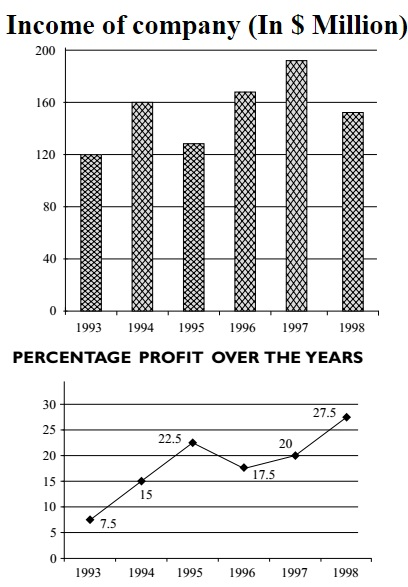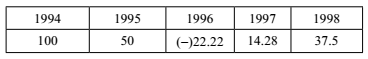## Introduction to Data Interpretation

#### Data Interpretation

Direction: Study the following graphs carefully and answer the questions given below:1. Approximately what was the expenditure in 1994?

1. Let us assume E was the expenditure in 1994.
As we can see in the graph given that,
Profit = 15 % ,
Income in 1994 = 160
Then according to question,
E + E x 15 % = 160

##### Correct Option: C

Let us assume E was the expenditure in 1994.
As we can see in the graph given that,
Profit = 15 % ,
Income in 1994 = 160
Then according to question,
E + E x 15 % = 160
E + E x 15 /100 = 160
(100E + E x 15 ) /100 = 160
115E / 100 = 160
115E = 160 x 100
E = 160 x 100 / 115
E = 139.13
Expenditure in 1994 E = 139.13 ≈ 140 Million

1. If the profit percentage in 1997 was 25, what would have been the expenditure in that year?

1. Let us assume E was the expenditure in 1997.
As we can see in the graph given that,
Income in 1997 = 190
Profit = 25 % (Given in the question.)
Then according to question,
E + E x 25 % = 190

##### Correct Option: D

Let us assume E was the expenditure in 1997.
As we can see in the given graph that,
Income in 1997 = 190
Profit = 25 % (Given in the question.)
Then according to question,
E + E x 25 % = 190
E + E x 25 /100 = 190
(100E + E x 25 ) /100 = 190
125E / 100 = 160
125E = 190 x 100
E = 190 x 100 / 125 = 190 x 4 / 5 = 38 x 4
E = 152
Expenditure in 1997 E = 152 Million

1. Approximately what was the average expenditure of the given years?

1. Total expenditure in 6 years = ( expenditure in 1993 + expenditure in 1994 + expenditure in 1995 + expenditure in 1996 + expenditure in 1997 + expenditure in 1998 ).
As we can see in the given graph that,
Income in 1993 = 120
Profit = 7.5 %
Then Expenditure in 1993 = 120 X 100 / 107.5
Similarly
Expenditure in 1994 = 160 X 100 / 115
Expenditure in 1995 = 130 X 100 / 122.5
Expenditure in 1996 = 170 X 100 / 117.5
Expenditure in 1997 = 190 X 100 / 120
Expenditure in 1998 = 150 X 100 / 127.5

##### Correct Option: B

Total expenditure in 6 years = ( expenditure in 1993 + expenditure in 1994 + expenditure in 1995 + expenditure in 1996 + expenditure in 1997 + expenditure in 1998 ).
As we can see in the given graph that,
Income in 1993 = 120
Profit = 7.5 %
Then Expenditure in 1993 = 120 X 100 / 107.5
Similarly
Expenditure in 1994 = 160 X 100 / 115
Expenditure in 1995 = 130 X 100 / 122.5
Expenditure in 1996 = 170 X 100 / 117.5
Expenditure in 1997 = 190 X 100 / 120
Expenditure in 1998 = 150 X 100 / 127.5
Total Expenditure in 6 years = ( 120 X 100 / 107.5 ) + ( 160 X 100 / 115 ) + ( 130 X 100/122.5 ) + ( 170 X 100 / 117.5 ) + ( 190 X 110 / 120 ) + ( 150 X 100/127.5 )
Total Expenditure in 6 years = 111.6 2 +139.13 + 106.16 + 144.68 + 158.33 + 117.64
Total Expenditure in 6 years = 777.56
Average expenditure per years = 777.56 / 6 = 12 .59 ≈ \$ 130 Million

1. In which of the following years was the amount of profit the maximum?

1. We can use the direct formula for

 Profit = Income x1 - 100100 + % profit

##### Correct Option: E

We can use the direct formulae for

 Profit = Income x1 - 100100 + % profit

Calculate the profit for each year n compare them.
We can see that the profit is maximum in 1998.

1. In which of the following years was the increase/ decrease in percent profit from the previous year the minimum?

1. We can use the direct formula for

 Loss = Income x1 - 100100 - % Loss

Calculate the Percent increase/decrease from the previous year.##### Correct Option: A

We can use the direct formula for

 Loss = Income x1 - 100100 - % Loss

Percent profit increase/decrease from the previous year